# Atomic Structure

## Exercise

#### Question 1

Fill in the blanks:

(a) Dalton said that ............... could not be divided.

(b) An ion which has a positive charge is called a ............... .

(c) The outermost shell of an atom is known as ............... .

(d) The ............... of an atom is very hard and dense.

(e) Neutrons are ............... particles having mass equal to that of protons.

(f) Isotopes are the atoms of ............... element having the ............... atomic number but ............... mass number.

(a) atoms

(b) Cation

(c) Valence shell

(d) nucleus

(e) neutral

(f) same, same, different

#### Question 2

Write True or False for the following statements:

(a) An atom on the whole has a positive charge.

(b) The maximum number of electrons in the first shell can be 8.

(c) The central part of an atom is called nucleus.

(a) False
Correct statement — An atom on the whole is electrically neutral.

(b) False
Correct statement — The maximum number of electrons in the first shell can be 2.

(c) True

#### Question 3

Give the following a suitable word/phrase.

(a) The subatomic particle with negative charge and negligible mass.

(b) Protons and neutrons present in the nucleus.

(c) The electrons present in the outermost shell.

(d) Arrangement of electrons in the shells of an atom.

(e) The number of protons present in the nucleus of an atom.

(f) The sum of the number of protons and neutrons of an atom.

(g) Atoms of same element with same atomic number but a different mass number.

(h) The smallest unit of an element which takes part in a chemical reaction.

(a) Electron

(b) Nucleons

(c) Valence electrons

(d) Electronic configuration

(e) Atomic number

(f) Mass number

(g) Isotopes

(h) Atom

#### Question 4a

The outermost shell of an atom is known as

1. Valency
2. Valence electrons
3. Nucleus
4. Valence shell

Valence shell

Reason — The outermost shell of an atom is known as its valence shell or valence orbit.

#### Question 4b

The number of valence electrons present in magnesium is

1. two
2. three
3. four
4. five

two

Reason — The atomic number of magnesium is 12. Its electronic configuration is 2, 8, 2. So two electrons are present in the outermost shell which are called valence electrons.

#### Question 4c

The subatomic particle with positive charge is

1. proton
2. neutron
3. electron
4. nucleon

proton

Reason — A proton is a positively charged particle present in the nucleus of an atom.

#### Question 4d

If the atomic number of an atom is 17 and mass number is 35, then number of neutrons will be

1. 35
2. 17
3. 18
4. 52

18

Reason — Number of neutrons = Mass number - Atomic number = 35-17 = 18.
So, number of neutrons = 18.

#### Question 4e

The number of electrons in an atom is equal to the number of

1. protons in a neutral atom
2. neutrons in a neutral atom
3. nucleons in a neutral atom
4. none of the above

protons in a neutral atom

Reason — An electrically neutral atom has an equal number of electrons and protons.

#### Question 4f

The sum of number of protons and number of neutrons present in the nucleus of an atom is called its

1. mass number
2. atomic number
3. number of electrons
4. all of the above

mass number

Reason — The sum of number of protons and number of neutrons present in the nucleus of an atom is called the mass number of that element.

#### Question 5

Name three fundamental particles of an atom. Give the symbol with charge on each particle.

The three fundamental particles of an atom are:

1. Electrons — An electron is negatively charged particle denoted by the symbol -1e0.
2. Protons — A proton is positively charged particle denoted by the symbol +1p1.
3. Neutrons — A neutron is neutral charged particle denoted by symbol 0n1.

#### Question 6

Define the following terms:

(a) Atomic number

(b) Mass number

(c) Nucleons

(d) Valence shell

(a) The number of protons present in the nucleus of the atom of an element is called its atomic number.

(b) The sum of the number of protons and the number of neutrons present in the nucleus of the atom of an element is called the mass number of that element.

(c) The protons and neutrons are collectively called as nucleons.

(d) The outermost shell of an atom is known as its valence shell or valence orbit.

#### Question 7

Mention briefly the salient features of Dalton's atomic theory (five points).

The salient features of Dalton's atomic theory are:

1. Matter consists of very small and indivisible particles called atoms.
2. Atoms can neither be created nor be destroyed.
3. Atoms of an element combine in small numbers to form molecules of that element.
4. Atoms of an element combine with the atoms of another element in a simple whole number ratio to form molecules of a compound.
5. Atoms are the smallest unit of matter that take part in chemical reactions during which only rearrangement of atoms takes place.

#### Question 8

(a) What are the two main features of Rutherford's atomic model?

(b) State its one drawback.

(a) The two main features of Rutherford's atomic model are:

1. An atom consists of mainly two parts : the centrally located nucleus and the outer circular orbits.
2. The nucleus is positively charged mass and the entire mass of the atom is concentrated on it. The size of the nucleus is very small and it is the densest part of the atom. Electrons revolve around the nucleus in circular orbits called shells. An atom is electrically neutral i.e., number of protons is equal to number of electrons.

(b) Rutherford could not explain the structural stability of an atom.

#### Question 9

What are the observations of the experiment done by Rutherford in order to determine the structure of an atom?

In his experiment, Rutherford bombarded a thin sheet of gold (of 0.00004 cm thickness) with fast-moving alpha particles in an evacuated chamber. He made the following observations from his experiment:

1. Most of the alpha particles passed straight through the foil without any deflection from their path.
2. A small fraction of them were deflected from their original path by small angles.
3. Only a few particles bounced back.

#### Question 10

State the mass number, the atomic number, number of neutrons and electronic configuration of the following atoms.

(a) 12C6

(b) 16O8

(c) 19F9

(d) 20Ne10

(e) 27Al13

(f) 35Cl17

Also, draw atomic diagrams for each of them.

(a) 12C6

Atomic number: 6

Mass number: 12

Number of neutrons: 12 - 6 = 6

Electronic configuration: 2, 4

Atomic diagram: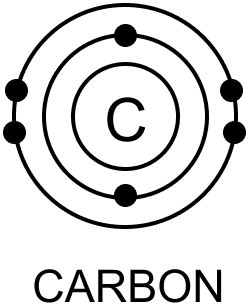(b) 16O8

Atomic number: 8

Mass number: 16

Number of neutrons: 16 - 8 = 8

Electronic configuration: 2, 6

Atomic diagram: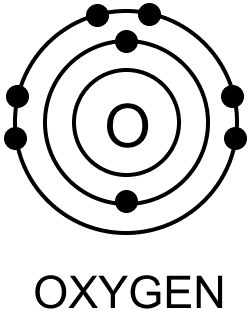(c) 19F9

Atomic number: 9

Mass number: 19

Number of neutrons: 19 - 9 = 10

Electronic configuration: 2, 7

Atomic diagram: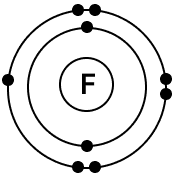(d) 20Ne10

Atomic number: 10

Mass number: 20

Number of neutrons: 20 - 10 = 10

Electronic configuration: 2,8

Atomic diagram: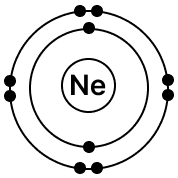(e) 27Al13

Atomic number: 13

Mass number: 27

Number of neutrons: 27 - 13 = 14

Electronic configuration: 2, 8, 3

Atomic diagram: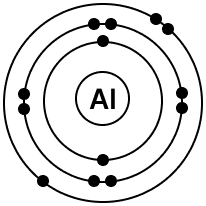(f) 35Cl17

Atomic number: 17

Mass number: 35

Number of neutrons: 35 - 17 = 18

Electronic configuration: 2, 8, 7

Atomic diagram: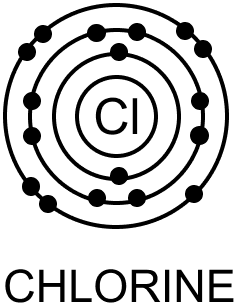#### Question 11

What is variable valency? Name two elements having variable valency and state their valencies.

Some elements exhibit more than one valency and they are said to have variable valency.

Examples: Iron and copper.

Iron (Fe)Ferrous, Ferric2, 3
Copper (Cu)Cuprous, Cupric1, 2

#### Question 12

The atomic number and the mass number of sodium are 11 and 23 respectively. What information is conveyed by this statement?

Atomic number of sodium is 11 means the number of protons present in the nucleus of sodium atom is 11 and the number of electrons present is 11. Mass number of sodium is 23 means the number of protons + number of neutrons is 23. So number of neutrons present is 12.

#### Question 13

Draw the diagrams representing the atomic structures of the following:

(a) Nitrogen

(b) Neon

(a) Atomic structure diagram of Nitrogen is given below: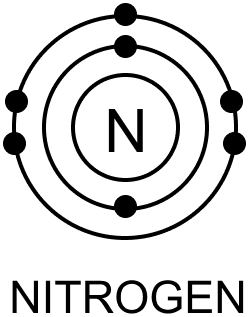(b) Atomic structure diagram of Neon is given below:#### Question 14

Explain the rule with example according to which electrons are filled in various energy levels.

Neil Bohr and Charles Bury proposed a scheme for distribution of electrons in different shells of an atom based on the formula 2n2, where n denotes the number of shell.

The following rules are followed for writing the number of electrons in different energy levels or shells:

1. The maximum number of electrons in each shell or orbit is determined by the formula 2n2, where n is the number of shell.
K shell, n=1, no. of electrons = 2 x 12 = 2
L shell, n=2, no. of electrons = 2 x 22 = 8
M shell, n=3, no. of electrons = 2 x 32 = 18
2. Electrons are not accommodated in a given shell, unless the inner shells are filled. Shells are filled in step-wise manner.
3. If the atom has only one shell, the outermost shell must have 2 electrons to achieve stable electronic configuration. This is called the duplet rule. If the atom has more than one shell, then its outermost shell must have 8 electrons to achieve stable electronic configuration. This is called the octet rule.

Example: Potassium has 19 electrons. The first shell will take 2 electrons, second shell will take 8 electrons. Remaining 9 electrons should be taken by third shell but according to octet rule, the outermost shell should not accommodate more than 8 electrons. So third shell take 8 electrons and remaining 1 electron will go to fourth shell. So electronic configuration of potassium is:

$\begin{matrix} \text{K} & \text{L} & \text{M} & \text{N} \\ 2 & 8 & 8 & 1 \end{matrix}$

#### Question 15

The atom of an element is made up of 4 protons, 5 neutrons and 4 electrons. What is its atomic number and mass number?

Atomic number = number of protons = 4.

Mass number = number of protons + number of neutrons = 4 + 5 = 9.

#### Question 16

(a) What are the two main parts of which an atom is made of?

(b) Where is the nucleus of an atom situated?

(c) What are orbits or shells of an atom?

(a) An atom is made of:

1. The nucleus
2. The orbits or the shells present in the empty space that surrounds the nucleus.

(b) The nucleus is present in the central part of an atom.

(c) Orbits or shells are the imaginary paths traced by the electrons in the empty space surrounding the nucleus.

#### Question 17

What are isotopes? How does the existence of isotopes contradict Dalton's atomic theory?

Isotopes are the atoms of the same element with the same atomic number but a different mass number due to the difference in the number of neutrons in their nucleus.

According to Dalton's theory, atoms of an element are identical in all respects like size, mass, density, chemical properties. But isotopes of an element have atoms that have same atomic number i.e. same number of protons and electrons but different mass number i.e. number of neutrons are different. So isotopes differ from each other in their physical properties like density, melting point, boiling point, etc. This is a contradiction to Dalton's atomic theory.

#### Question 18

Complete the table below by identifying A, B, C, D, E and F.

ElementSymbolNumber of protonsNumber of neutronsNumber of electrons
Fluorine9F199AB
AluminiumCD1413
Potassium19K39EF19

A → 10

B → 9

C → 13Al27

D → 13

E → 19

F → 20

Completed table is shown below:

ElementSymbolNumber of protonsNumber of neutronsNumber of electrons
Fluorine9F199109
Aluminium13Al27131413
Potassium19K39192019